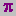Compute ()

Controller > compute new column of data

The point of it

This function brings up a calculator, where you can choose functions to calculate values which interest you. For example, a word list routinely provides the frequency of each type, and that frequency as a percentage of the overall text tokens. You might want to insert a further column showing the frequency as a percentage of the number of word types, or a column showing the frequency as a percentage of the number of text files from which the word list was created.

How to do it

Just press Compute | New Column and create your own formula. You'll see standard calculator buttons with the numbers 0 to 9, decimal point, brackets, 4 basic functions. To the right there's a list of standard mathematical functions to use (pi, square root etc.): to access these, double-click on them. Below that you will see access to your own data in the current list, listing any number-based column-headings. You can drag or double-click them too.

Absolute and Relative

Your own data can be accessed in two ways. A relative access (the default) means that as in a spreadsheet you want the new column to access data from another column but in the same row. Absolute access means accessing a fixed column and row.

Examples

 Rel(2) ÷ 5 for each row in your data, the new column will contain the data from column 2 of the same row, divide it by 5, and put the result in your new column.
 RelC(2) for each row in your data, the new column will contain the data from column 2 of the same row, add it to a running total, and put the result in your new column.
 Rel(3) + (Rel(2) ÷ 5) for each row in your data, the new column will contain the data from column 2 of the same row, divide it by 5, add it to the data from column 3 of the same row, and put the result in your new column.
 Abs(2;1) ÷ 5 for each row in your data, the new column will contain the data from column 2 of row 1, divide it by 5, and put the result in your new column. This example is just to illustrate; it would be silly as it would give the exact same result in every row.
 Rel(2) ÷ Abs(2;1) × 100 for each row in your data, the new column will contain the data from column 2 of the same row, divide it by column 2 of row 1 and multiply it by 100, putting the result in your new column. This would give column 3 as a percentage of the top result in column 2. For the first row it'd give 100%, but as the frequencies declined so would their percentage of the most frequent item.

You can format (or even delete) any variables computed in this way: see layout.